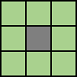# bwareafilt

## 语法

``BW2 = bwareafilt(BW,range)``
``BW2 = bwareafilt(BW,n)``
``BW2 = bwareafilt(BW,n,keep)``
``BW2 = bwareafilt(___,conn)``

## 说明

``BW2 = bwareafilt(BW,range)` 从二值图像 `BW` 中提取对象面积在指定 `range` 内的所有连通分量（对象），并生成另一个二值图像 `BW2`。`bwareafilt` 返回仅包含符合条件的那些对象的二值图像 `BW2`。`

``BW2 = bwareafilt(BW,n)` 保留 `n` 个最大对象。如果第 `n` 个位置出现结值，则 `BW2` 中仅包含前 `n` 个对象。`
``BW2 = bwareafilt(BW,n,keep)` 指定是保留 `n` 个最大对象还是 `n` 个最小对象。`
``BW2 = bwareafilt(___,conn)` 指定定义对象的像素连通性。`

## 示例

`BW = imread('text.png');`

`BW2 = bwareafilt(BW,[40 50]);`

`imshowpair(BW,BW2,'montage')``BW = imread('text.png');`

`BW2 = bwareafilt(BW,5);`

`imshowpair(BW,BW2,'montage')`## 输入参数

`4``8`